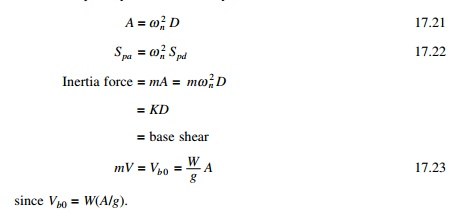Home | | Structural Dynamics and Earthquake Engineering | Pseudo-spectral acceleration

# Pseudo-spectral acceleration

The parameters have certain characteristics that are of practical interest.

Pseudo-spectral acceleration

Consider a quantity A for a SDOF systemLet A/g be called base shear coefficient or lateral force coefficient. It is used in building codes to represent the coefficient by which structural weight is multiplied to obtain the base shear. A is the peak pseudo-acceleration. A pseudo-acceleration response spectrum is a plot of A as functions of Tn.

The parameters have certain characteristics that are of practical interest. The pseudo-spectral velocity Spv is close to spectral velocity Sv for short period structures and is almost equal to the intermediate periods but is different for long period structures. A comparison of the NS component of the Northridge earthquakes is made in Fig. 17.14 for spectral velocity and spectral acceleration for Ď‚ = 0.05. For zero damping, pseudo-spectral acceleration is identical to spectral acceleration Sa. However, for damping other than zero these two are slightly different. Nevertheless, for damping levels encountered in most engineering applications the two may be considered to be practically equal.

Evaluation of spectral displacement Sd by the use of Eq. 17.9a after numerical integration of Eq. 17.2 is to be carried out to obtain the time history response u(t). The corresponding pseudo-spectral velocity Spv and pseudo-spectral acceleration Spa can readily be established from Eq. 17.19 and 17.21.Study Material, Lecturing Notes, Assignment, Reference, Wiki description explanation, brief detail
Civil : Structural dynamics of earthquake engineering : Pseudo-spectral acceleration |